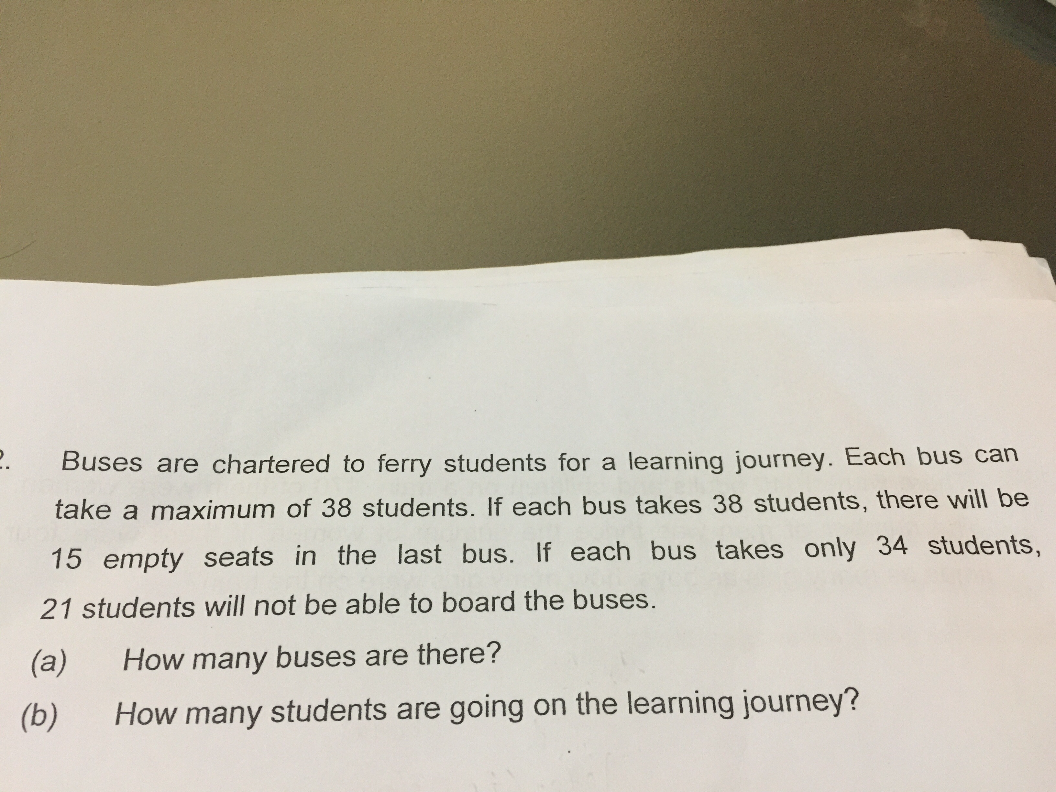# QuestionHi can help me with this question. Thks

There were a total of u buses.

Scenario 1

If all buses took 38 students, last bus would have 15 empty seats.

Total number of students: 38u – 15

Scenario 2

If all buses took 34 students, 21 students would not be able to board.

Total number of students: 34u + 21

38u – 15 = 34u + 21

4u = 36

u = 9

(a) There were 9 buses

(b) There were 34u + 21 = 327 students

0 Replies 2 Likes ✔Accepted Answer

(a)
(number of buses x 38) – 15 ——- (number of buses x 34) + 21
number of buses ——- (21 + 15)/(38 – 34) = 9

(b)
9 x 38 – 15 = 327 or 9 x 34 + 21 = 327

Ans : (a) 9 buses; (b) 327 students.

0 Replies 0 Likes

After the students were seated with 38 in each bus, a teacher saw some 15 seats left and picked out 4 students from each bus (=38-34) to fill up these 15 seats but left with 21 students without seats.  🙁

Total students picked out = 15+21=36

No. of buses = 36/4= 9 buses#

Total no. of students = 9 x 38 – 15 = 327# (also equals to 9 x 34 + 21)

*see how poor in Maths the teacher is… she should only pick out 1 student from each of the 9 buses to fill up 9 seats and left 6 empty seats. If she picks out 2 students from each of the 9 buses, 3 poor students will be left in school and can’t go to the learning journey…

Have fun to let your child think more to truely understand each scenario, better than touch and go for each correct answer.  🙂